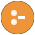Did you know ... Search Documentation:Title for pldoc(dir_index)

# clp

## Prolog files

bounds.pl  -- Simple integer solver that keeps track of upper and lower boundsclp_events.plclpb.pl  -- CLP(B): Constraint Logic Programming over Boolean Variableslabeling/1Enumerate concrete solutions.random_labeling/2Select a single random solution.sat/1True iff Expr is a satisfiable Boolean expression.sat_count/2Count the number of admissible assignments.taut/2Tautology check.weighted_maximum/3Enumerate weighted optima over admissible assignments.clpfd.pl  -- CLP(FD): Constraint Logic Programming over Finite Domains#/\/2P and Q hold.#</2The arithmetic expression X is less than Y.#<==/2Q implies P.#<==>/2P and Q are equivalent.#=/2The arithmetic expression X equals Y.#=</2The arithmetic expression X is less than or equal to Y.#==>/2P implies Q.#>/2Same as Y #< X.#>=/2Same as Y #=< X.#\/1Q does not hold.#\/2Either P holds or Q holds, but not both.#\//2P or Q holds.#\=/2The arithmetic expressions X and Y evaluate to distinct integers.all_different/1Like all_distinct/1, but with weaker propagation.all_distinct/1True iff Vars are pairwise distinct.automaton/3Describes a list of finite domain variables with a finite automaton.automaton/8Describes a list of finite domain variables with a finite automaton.chain/2Zs form a chain with respect to Relation.circuit/1True iff the list Vs of finite domain variables induces a Hamiltonian circuit.cumulative/1Equivalent to `cumulative(Tasks, [limit(1)])`.cumulative/2Schedule with a limited resource.disjoint2/1True iff Rectangles are not overlapping.element/3The N-th element of the list of finite domain variables Vs is V.empty_fdset/1Set is the empty FD set.empty_interval/2Min..Max is an empty interval.fd_degree/2Degree is the number of constraints currently attached to Var.fd_dom/2Dom is the current domain (see in/2) of Var.fd_inf/2Inf is the infimum of the current domain of Var.fd_set/2Set is the FD set representation of the current domain of Var.fd_size/2Reflect the current size of a domain.fd_sup/2Sup is the supremum of the current domain of Var.fd_var/1True iff Var is a CLP(FD) variable.fdset_add_element/3Set2 is the same FD set as Set1, but with the integer Elt added.fdset_complement/2The FD set Complement is the complement of the FD set Set.fdset_del_element/3Set2 is the same FD set as Set1, but with the integer Elt removed.fdset_disjoint/2The FD sets Set1 and Set2 have no elements in common.fdset_eq/2True if the FD sets Set1 and Set2 are equal, i.fdset_intersect/2The FD sets Set1 and Set2 have at least one element in common.fdset_intersection/3Intersection is an FD set (possibly empty) of all elements that the FD sets Set1 and Set2 have in common.fdset_interval/3Interval is a non-empty FD set consisting of the single interval Min..Max.fdset_max/2Max is the upper bound (supremum) of the non-empty FD set Set.fdset_member/2The integer Elt is a member of the FD set Set.fdset_min/2Min is the lower bound (infimum) of the non-empty FD set Set.fdset_parts/4Set is a non-empty FD set representing the domain Min..Max \/ Rest, where Min..Max is a non-empty interval (see fdset_interval/3) and Rest is another FD set (possibly empty).fdset_singleton/2Set is the FD set containing the single integer Elt.fdset_size/2Size is the number of elements of the FD set Set, or the atom sup if Set is infinite.fdset_subset/2The FD set Set1 is a (non-strict) subset of Set2, i.fdset_subtract/3The FD set Difference is Set1 with all elements of Set2 removed, i.fdset_to_list/2List is a list containing all elements of the finite FD set Set, in ascending order.fdset_to_range/2Domain is a domain equivalent to the FD set Set.fdset_union/2The FD set Union is the n-ary union of all FD sets in the list Sets.fdset_union/3The FD set Union is the union of FD sets Set1 and Set2.global_cardinality/2Global Cardinality constraint.global_cardinality/3Global Cardinality constraint.in/2Var is an element of Domain.in_set/2Var is an element of the FD set Set.indomain/1Bind Var to all feasible values of its domain on backtracking.ins/2The variables in the list Vars are elements of Domain.is_fdset/1Set is currently bound to a valid FD set.label/1Equivalent to `labeling([], Vars)`.labeling/2Assign a value to each variable in Vars.lex_chain/1Lists are lexicographically non-decreasing.list_to_fdset/2Set is an FD set containing all elements of List, which must be a list of integers.range_to_fdset/2Set is an FD set equivalent to the domain Domain.scalar_product/4True iff the scalar product of Cs and Vs is in relation Rel to Expr.serialized/2Describes a set of non-overlapping tasks.sum/3The sum of elements of the list Vars is in relation Rel to Expr.transpose/2Transpose a list of lists of the same length.tuples_in/2True iff all Tuples are elements of Relation.zcompare/3Analogous to compare/3, with finite domain variables A and B.clpq.plclpr.plinclpr.plsimplex.pl  -- Solve linear programming problemsassignment/2Solves a linear assignment problem.constraint/3Adds a linear or integrality constraint to the linear program corresponding to state S0.constraint/4Like constraint/3, and attaches the name Name (an atom or compound term) to the new constraint.constraint_add/4Left is a list of `Coefficient*Variable` terms.gen_state/1Generates an initial state corresponding to an empty linear program.maximize/3Maximizes the objective function, stated as a list of `Coefficient*Variable` terms that represents the sum of its elements, with respect to the linear program corresponding to state S0.minimize/3Analogous to maximize/3.objective/2Unifies Objective with the result of the objective function at the obtained extremum.shadow_price/3Unifies Value with the shadow price corresponding to the linear constraint whose name is Name.transportation/4Solves a transportation problem.variable_value/3Value is unified with the value obtained for Variable.